# SUMIF With Multiple CriteriaArticle byExcelMojo Team## SUMIF And SUMIFS Function With Multiple Criteria?

The SUMIF and SUMIFS functions with multiple criteria are inbuilt Math & Trig functions. They determine the sum of the cell values based on the specified multiple conditions.

Users can use the SUMIF with multiple criteria to find the sum of the data values in a row or column with multiple conditions. However, they can apply the SUMIFS() to determine the sum of the given cell range based on checking the specified conditions.

For example, the following image shows three tables.

• The first table contains an item list, categories, and quantity details.
• The second table contains a specific item and its category.
• In the third table, we will determine the quantity value based on the specified details in the second table and the source data in the first table and display the output.

Enter the formula =SUMIF(A2:A11,F1,C2:C11)+SUMIF(B2:B11,F2,C2:C11) in cell F5, and =SUMIFS(C2:C11,A2:A11,F1,B2:B11,F2) in cell F6. Here, we use SUMIF with multiple criteria to get the result for the same conditions. The output is shown in cells F5 and F6.

[Note: Column G is for our reference].

[Output Observation: The expression for SUMIF with multiple criteria in cell F5 contains two SUMIF excel functions. While the first one returns the sum of all quantity values for Item 1, the second SUMIF() determines the sum of all quantity values for Category A. And thus, the formula adds the two values to return the total quantity, 18000.

However, in the case of the SUMIFS with multiple criteria, cell F6 shows the result as 7600. The reason is that the SUMIFS excel function checks the two specified criteria and returns the sum of all quantity values of Item 1 in Category A. And as rows 2, 6, and 11 satisfy the two conditions, the function adds the corresponding quantity values to return the total quantity as 7600.]

###### Key Takeaways
• The SUMIF and SUMIF functions with multiple conditions add the value of cells satisfying the given criteria.
• Users can use the function SUMIF with multiple criteria when adding a row or column based on the given conditions.
• We can achieve SUMIF with multiple conditions using the SUMIF() with SUM() and SUMPPODUCT().
• Use the SUMIFS with multiple criteria when finding the sum of cell values, with the multiple conditions checked simultaneously. And the function SUMIFS with multiple criteria can be in the same column, different columns, or along a column and row.

### How to Use SUMIF Function with Multiple Criteria?

The steps to use the SUMIF with Multiple Criteria are as follows;

• 1: Choose an empty cell for the output.
• 2: Type =SUMIF( select the cell range, enter the first criteria as a cell value or a reference, enter the sum range(optional), and close the brackets.
• 3: Then press the “+”, and repeat step 2 with new values.
• 4: Type =SUMIF( select the second cell range, enter the required criteria as a cell value or a reference, enter the sum range(optional), and close the brackets.
• 5: The formula will be as per the syntax =SUMIF(range,criteria,[sum_range])+SUMIF(range,criteria,[sum_range]), and finally, press “Enter”.

#### Basic Example

We will determine the total sales figures of Dealers 1 and 2 in 2021 using SUMIF with multiple criteria.

Here, the first table contains the 2019-21 sales details of three dealers.

The steps to calculate using SUMIF with multiple criteria are,

1. Select cell B14, and set the Number Format in the Home tab as Currency.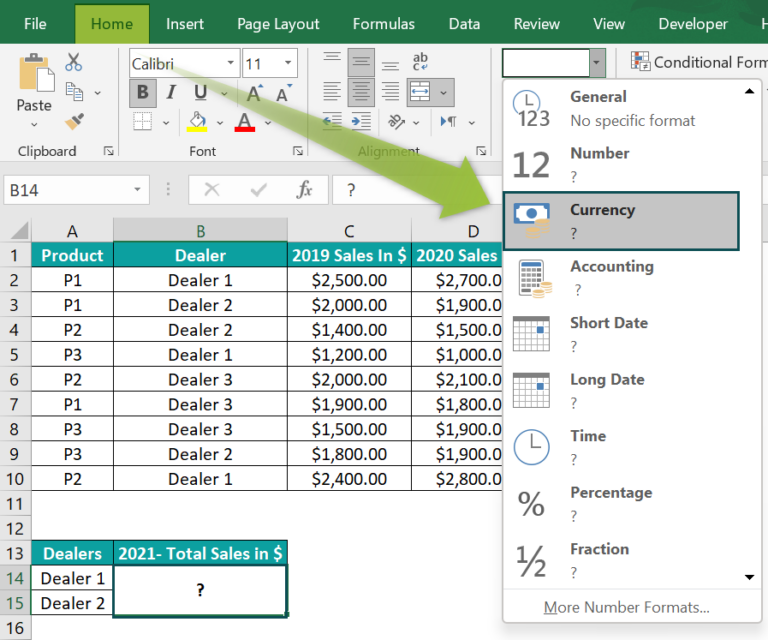The “Number Format” is changed to “Currency”, as shown below.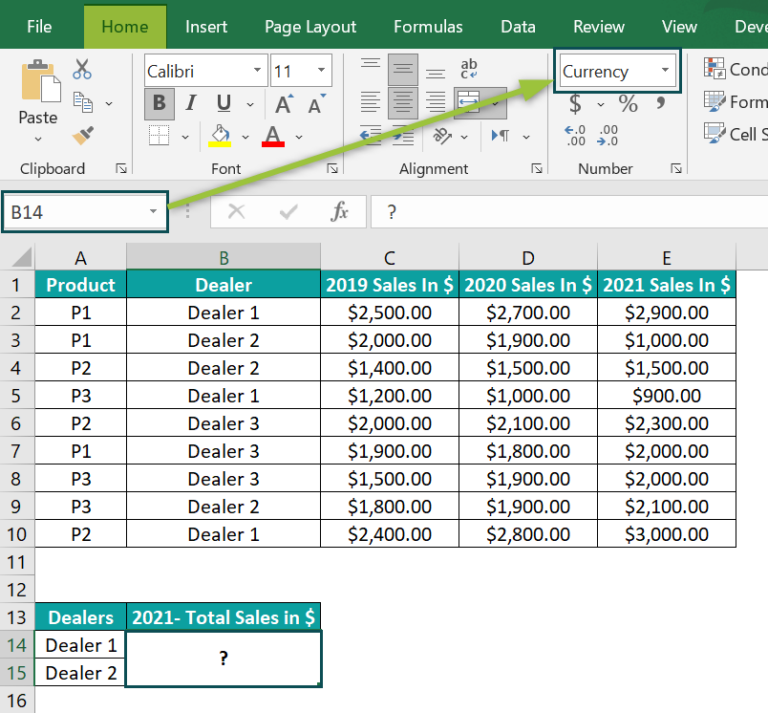2. Now, in cell B14, enter the formula=SUMIF(B2:B10,A14,E2:E10)+SUMIF(B2:B10,A15,E2:E10), and press Enter. The result is \$11,400, as shown below.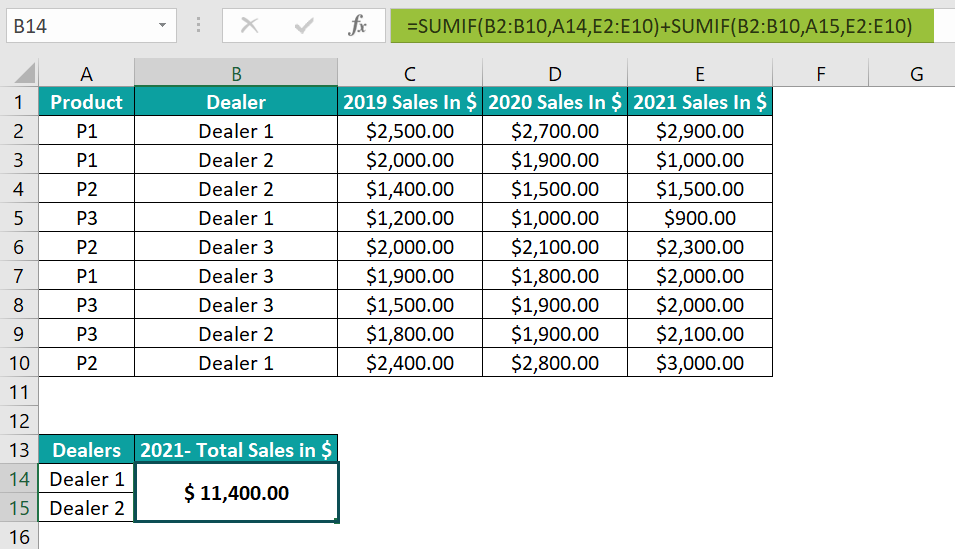[Alternatively, we can use the SUMIF() from the Formulas tab. And for that, we must select the target cell B14 and go to FormulasMath & TrigSUMIF to open the Function Arguments window.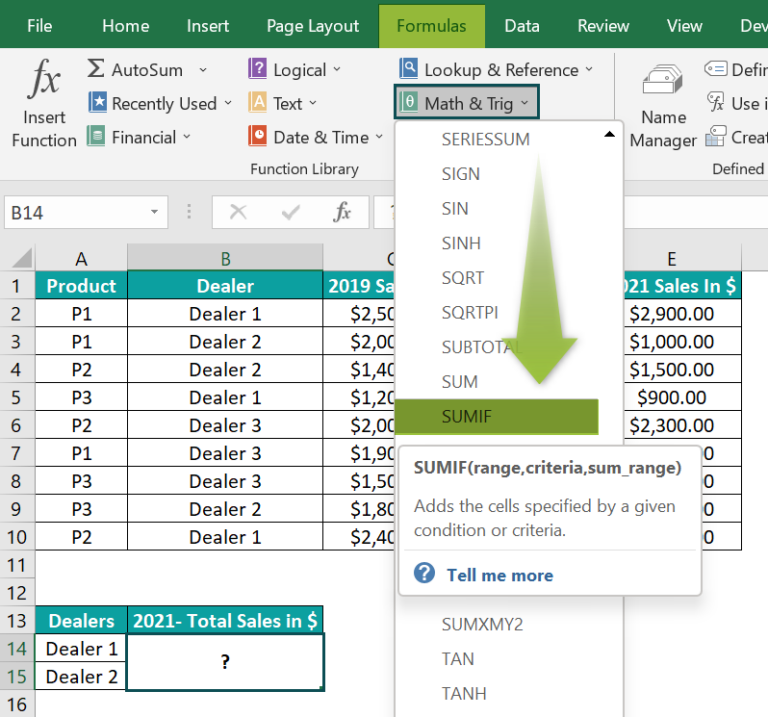In the Function Arguments window, enter the arguments in the “Range, Criteria, and Sum_range” fields, and click “OK”, as shown below.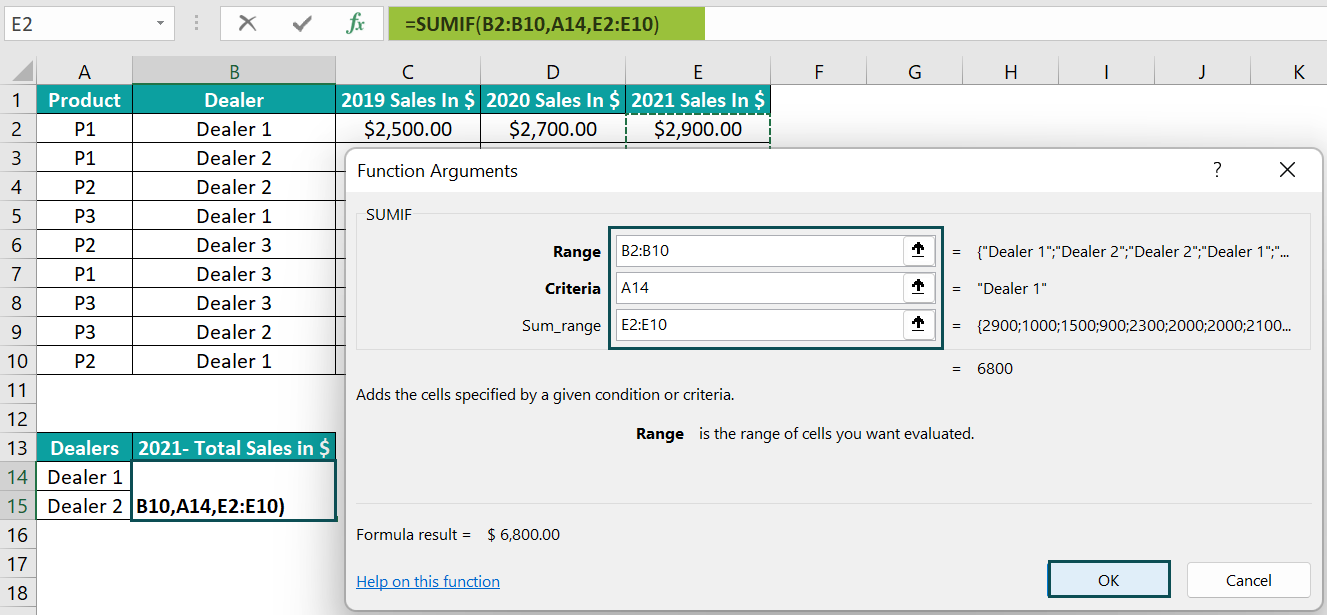In continuation with the formula, enter the ‘+’ symbol in the Formula Bar.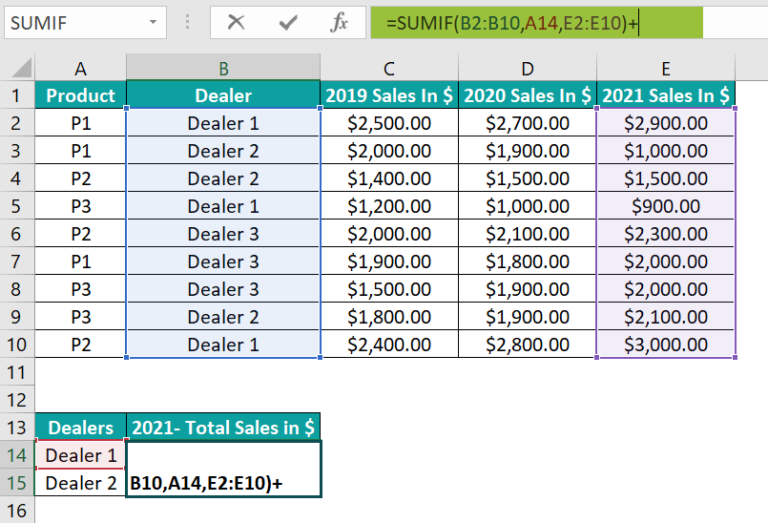Then, go to FormulasMath & TrigSUMIF to open the Function Arguments window again.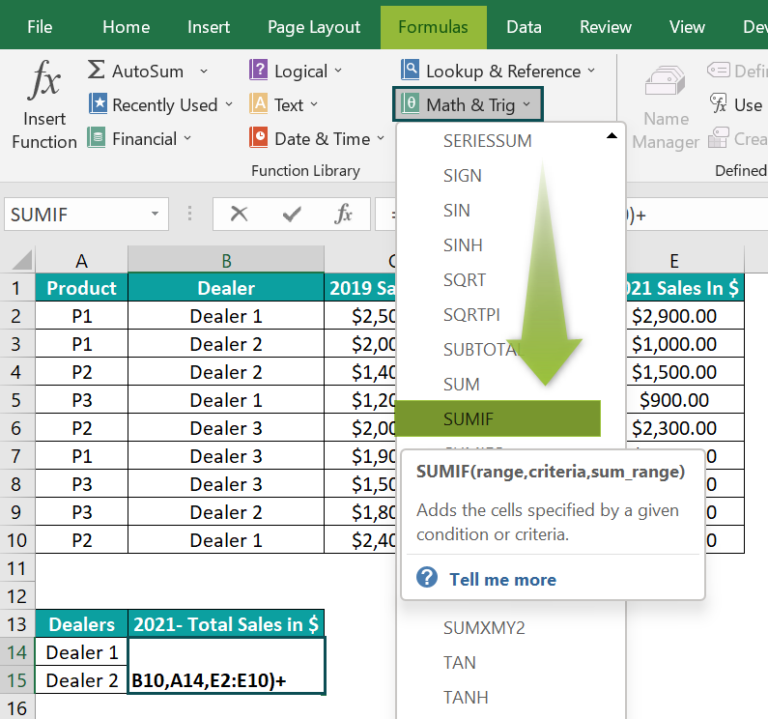Enter the second SUMIF() argument values in the Function Arguments window.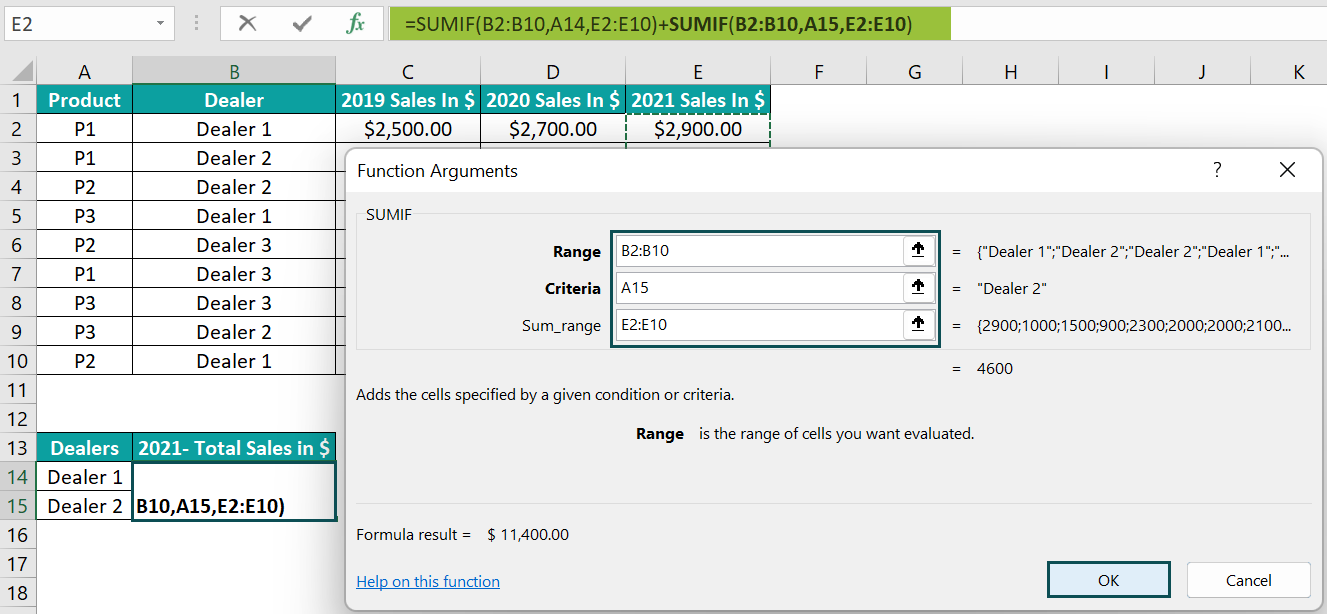And once we click OK, the complete formula gets executed to return the total sales value, \$11,400. In the above example, the first and second SUMIF functions determine the total 2021 sales figures of Dealers 1 and 2, respectively. And the formula adds the two function values to return the required output.]

### Examples of SUMIF Function with Multiple Criteria

We will understand some advanced scenarios with SUMIF Function With Multiple Criteria examples.

#### Example #1

We will determine the total number of units ordered for Samsung and Apple smartphones involving partial matches using the SUMIF with multiple criteria.

The first table shows a list of smartphone models with their delivery date and units ordered data.

The procedure to calculate using the SUMIF with multiple criteria is,

Select cell F2, enter the formula =SUMIF(A2:A9,”*Samsung*”,C2:C9)+SUMIF(A2:A9,”*Apple*”,C2:C9), and press Enter.

We get the result 1665, as shown above.

[Output Observation: The first SUMIF() checks for the value Samsung in the criteria range A2:A9, and cells A3, A5, and A9 satisfy the criteria through a partial match. And so, the corresponding sum_range cell values (cells C3, C5, and C9), 300, 265, and 210, get added.

Likewise, the second SUMIF() checks for the value Apple in the given criteria range A2:A9, and cells A2, A4, and A6 satisfy the criteria through a partial match. So, the function adds the corresponding sum_range cell values (cells C2, C4, and C6) 350, 290, and 250.

And finally, the formula adds the two SUMIF() return values to get the total number of units ordered for Samsung and Apple together, 1665.]

#### Example #2

We will calculate the total units of Grades 1, 2, and 3 using the SUM and SUMIF with multiple criteria.

The following table contains a list of fruits, their grades, and their delivery details.

The procedure to calculate using the SUM and the SUMIFS with multiple criteria is,

We get the result 13100, as shown above.

[Output Observation: We supply the criteria argument to the SUMIF() as an array, {“Grade 1”,”Grade 2”,”Grade 3”}. And the SUMIF() checks for the three grades in the criteria range B2:B11. It finds the required grades in cells B2, B3, B4, B6, B7, B9, and B11. Thus, it returns the units delivered from the corresponding column C cells, the sum_range.

Finally, the SUM() adds the total units of Grade 1, 2, and 3 fruits delivered in the seven cells mentioned above, 13100.]

#### Example #3

We will determine the total game points achieved using the SUMPRODUCT() and SUMIF with multiple criteria.

The first table contains a list of employees, their designations, and their game points details, and the second table contains specific designations.

The procedure to calculate using the SUMPRODUCT() and the SUMIF with multiple criteria is,

Select cell G3, enter the formula =SUMPRODUCT(SUMIF(B2:B11,G1:G2,C2:C11)), and press Enter.

We get the result 555, as shown above.

[Output Observation: First, the SUMIF() checks for the designations, Engineer and Senior Engineer, in the criteria range B2:B11. Then, it finds them in cells B2:B5 and B7:B9. And thus, it calculates the total game points scored by all the Engineers and Senior Engineers, 325 and 230, respectively.

Finally, the SUMPRODUCT() determines the sum of the values resulting from the SUMIF() and returns 555 as the total game points the Engineers and Senior Engineers scored.]

### How to Use SUMIFS Excel Function With Multiple Criteria

The steps to use the SUMIFS with Multiple Criteria are as follows;

• 1: Choose an empty cell for the output.
• 2: Type =SUMIFS( and select the cell range and enter the first criteria, second cell range, next required criteria, and so on… as a cell value or a reference, and close the brackets.
• 3: The formula will be as per the syntax =SUMIFS(sum_range,criteria_range1,criteria1,[criteria_range2,criteria2],…), and press “Enter”.

We will calculate the total points earned by two given students in the selected subject using SUMIFS Excel Function With Multiple Criteria.

The first table contains a list of students and the details of the points they earned in different subjects.

The procedure to calculate using the SUMIFS with multiple criteria is,

Select cell G4, enter the formula,

=SUMIFS(C2:C11,B2:B11,G1,A2:A11,G2)+SUMIFS(C2:C11,B2:B11,G1,A2:A11,G3), and press Enter.

We get the result 71, as shown below.

[Alternatively, we can apply the SUMIFS() using the option in the Formulas tab. And the steps are:

• Step 1: Select cell G4 and go to FormulasMath & TrigSUMIFS to open the Function Arguments window.
• Step 2: Enter the first SUMIFS() argument values. Once we click the second argument field to enter the value, we will get the next argument field.

Once we enter all the criteria for the first SUMIFS(), click OK in the Function Arguments window.

• Step 3: In continuation with the formula, click the Formula Bar, and enter a ‘+’.

Then, go to FormulasMath & TrigSUMIFS to open the Function Arguments window again and enter the second SUMIFS().

• Step 4: Enter the second SUMIFS() argument values in the Function Arguments window.

Finally, click OK to view the result.]

[Output Observation: The first SUMIFS() checks for a match where the student is Anita Keller and the subject is Mathematics in the Student and Subject columns, respectively. Rows 2 and 10 satisfy the given conditions. And thus, the function returns the sum of the values in the respective cells in column Points Earned (cells C2 and C10), 41. Likewise, the second SUMIFS() performs a similar check, except the student is Pat George. In this case, row 5 satisfies the condition. And so, the function returns the values in the corresponding cell in column Points Earned (cell C5), 30.

Finally, the formula adds the two SUMIFS functions’ output to return the value of 71.]

### Examples of SUMIFS Excel Function with Multiple Criteria

We will understand some advanced scenarios with SUMIFS Function With Multiple Criteria examples.

#### Example #1

We will calculate the total expenses in Q2s and Q3s using the SUMIFS with multiple criteria in same column.

The first table shows the quarterly expenses data for 2019-21.

The steps to calculate using the SUMIFS with multiple criteria in same column are,

• Step 1: Select cell E2 and set the Number Format option in the Home tab as Currency.

The “Number Format” is changed to “Currency”, as shown below.

• Step 2: Select cell E2, enter the formula =SUM(SUMIFS(B2:B13,A2:A13,{“Q2*”,”Q3*”})), and press Enter.

We get the result \$104,000, as shown above.

[Output Observation: First, the SUMIFS() finds the partial matches for the criteria values as an array.

While cells A3, A7, and A11 satisfy the condition for Q2, cells A4, A8, and A12 meet the Q3 criteria. Thus the SUMIFS() output is the sum of the expenses in all Q2s and Q3s, respectively, {62000,42000}.

And finally, the SUM() adds the two values to return the total expenses in all Q2s and Q3s as \$104,000.]

#### Example #2

We will determine the total assets ordered by two POCs, Angel Phelps and Stewart White, during Feb and Mar using the SUMIFS with multiple criteria in different columns.

The following table shows the data of assets ordered for different departments in an office.

The procedure to calculate using the SUMIFS with multiple criteria in different columns is,

Select cell B14, enter the formula =SUM(SUMIFS(D2:D11,C2:C11,{“Angel Phelps”,”Stewart White”},E2:E11,”>=2/1/2022″,E2:E11,”<=3/31/2022″)), and press Enter.

The result is 56, as shown above.

[Output Observation: The first criterion in the SUMIFS() is an array. So, the SUMIFS() finds cells in the POC column where the POC is Angel Phelps. And it checks for cells in the Order Date column where the dates fall in the required duration, Feb and Mar. So, row 5 meets the given criteria.

Likewise, the SUMIFS() performs a similar check for the POC, Stewart White. And rows 4 and 6 satisfy the specified criteria. Thus, the SUMIFS() output will be {20,36}, where the value 20 is the cell A5 value. And the value 36 is the sum of the values in cells C4 and C6, 21, and 15.

Finally, the SUM() adds the two values, 20 and 36, to return the total units ordered, based on the given criteria, 56.]

#### Example #3

We will calculate the total value of the orders not delivered using SUMIFS with multiple criteria SUMIFS. Also, we will understand how the function behaves when the source data contains empty cells.

The table below shows a list of products and their order data. Some of the date values are missing from the source data.

The steps to calculate using the SUMIFS with multiple criteria are,

• Step 1: Select cell B13 and set the Number Format option in the Home tab as Currency.

The “Number Format” is changed to “Currency”, as shown below.

• Step 2: Select cell B13, enter the formula =SUMIFS(D2:D11,B2:B11,”<>”,C2:C11,””), and press Enter.

We get the result \$44,000.00, as shown above.

[Output Observation: As we need the value of the orders not delivered, we need to check two criteria. The first one is that the order date cell for a product should not be blank, and the delivery date cell should be blank.

So the SUMIFS() checks the Order Date column for non-blank cells and the Delivery Date column for blank cells. Rows 7, 9, and 11 satisfy the conditions. And thus, the function adds the order values in the corresponding cells, D7, D9, and D11, to return the total value of orders not delivered as \$44,000.]

### Important Things to Note

• The SUMIF() checks one criterion at a time. So, when we have to use the function SUMIF with multiple criteria, the straightforward way to achieve the result is by using multiple SUMIF functions.
• The SUMIF() with multiple criteria will give an error if the data format is uneven. And if no cells meet the conditions, then the SUMIF() output will be 0.
• When using logical operators or wildcard characters in the SUMIF and SUMIFS functions, ensure we enclose them in double quotations.

1. Can we SUMIF with two criteria?

We can SUMIF with two criteria using the SUMIF() within the SUM().
Consider an example where the table contains a list of items, their brand, and total unit details.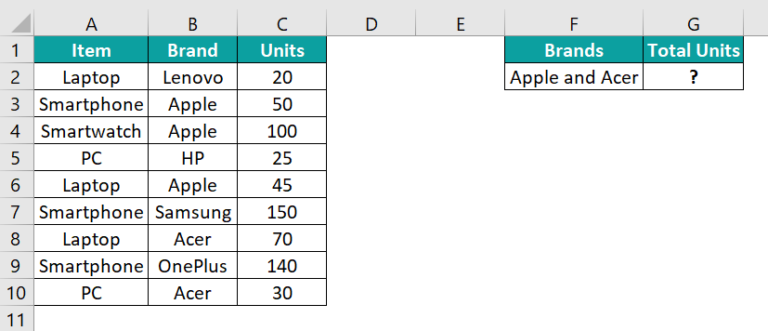The procedure to determine the total units sold for the brands specified using the SUMIF within SUM is,

Select cell G2, enter the formula =SUM(SUMIF(B2:B10,{“Apple”,”Acer”},C2:C10)), and press Enter.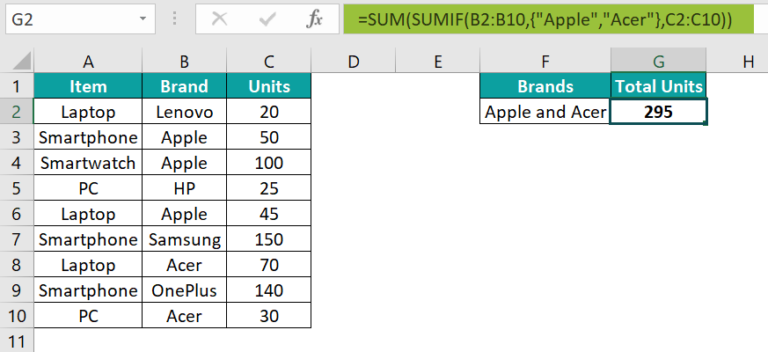The result is 295, as shown above.

[Output Observation: The SUMIF() checks for the Apple brand in the Brand column and adds the units in the corresponding cells in the Units column (cells C3, C4, and C6) to get 195. It repeats the same process for the Acer brand and adds the units in the corresponding cells in the Units column (cells C8 and C10) to get 100. The function returns these values as an array, {195,100}, as the criteria argument is an array, representing the two criteria.
And finally, the SUM() adds the two values in the SUMIF() array output to get the total units of the Apple and Acer brands as 295.]

2. Why is the SUMIFS function with multiple criteria not working?

The SUMIFS function with multiple criteria may not work for the following reasons:
We provided an inaccurate criteria range.
If we inserted values with the incorrect data format.
We used incorrect syntax order.

3. What is the difference between the SUMIF and SUMIFS with multiple criteria?

The difference between the SUMIF and SUMIFS with multiple criteria is that the SUMIF() adds a row or column by checking each condition one at a time. On the other hand, SUMIFS() adds a cell range by checking all the conditions simultaneously.